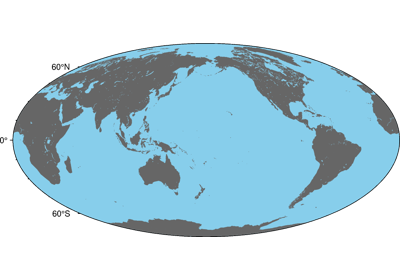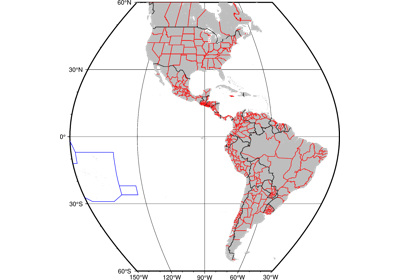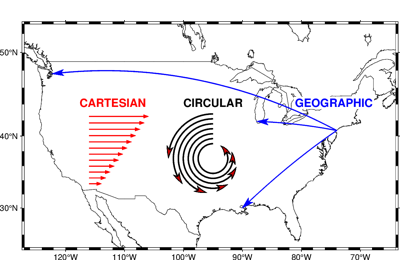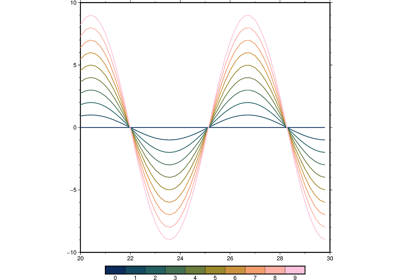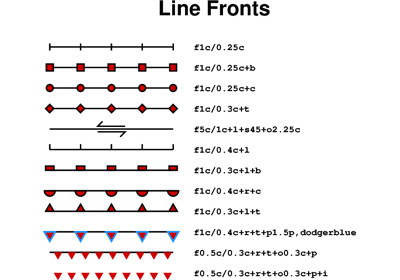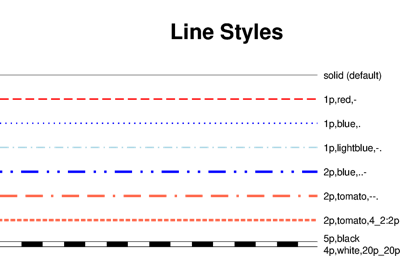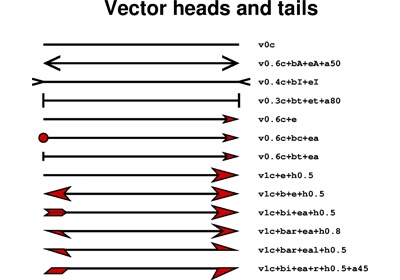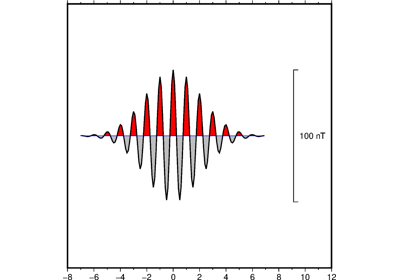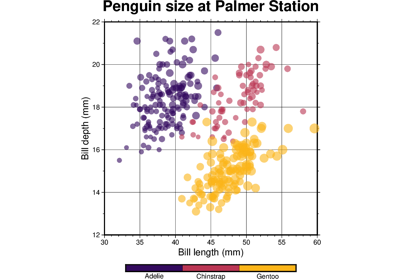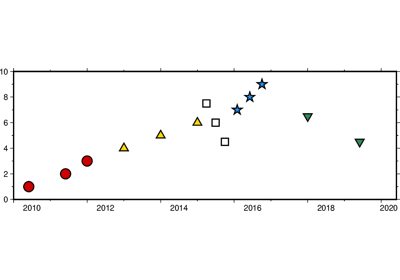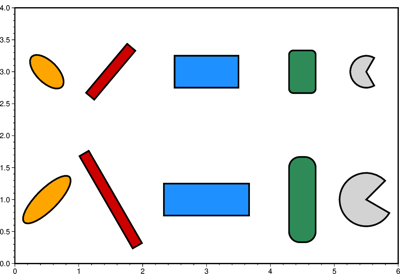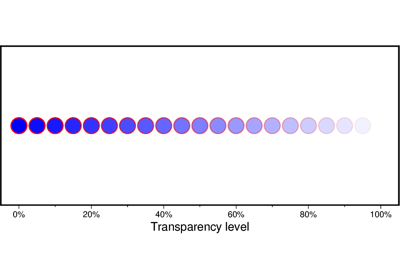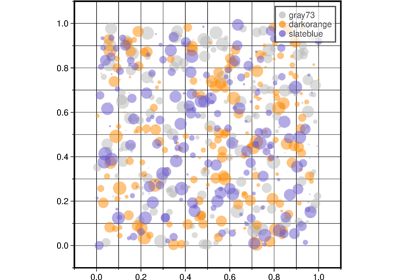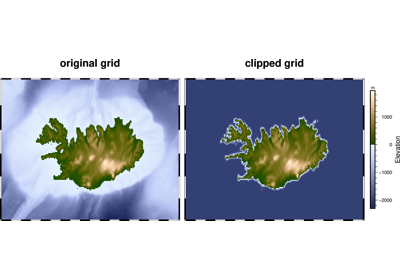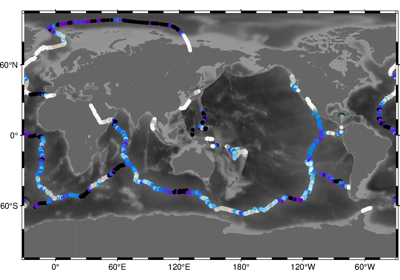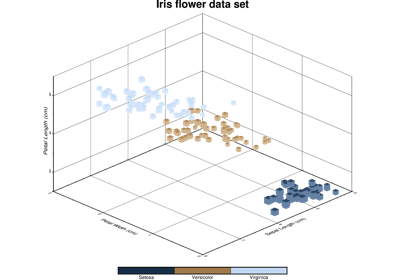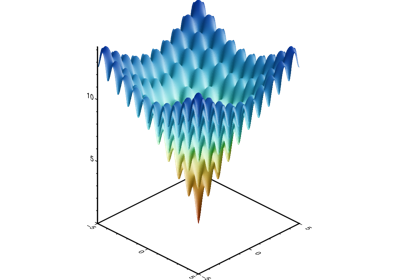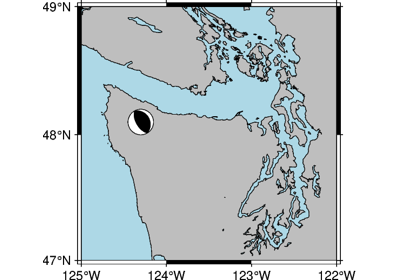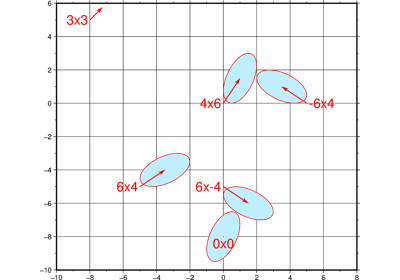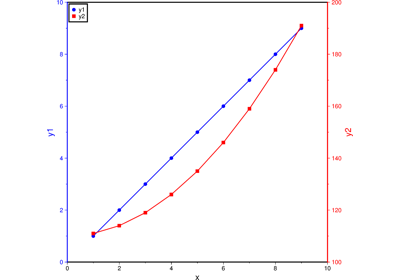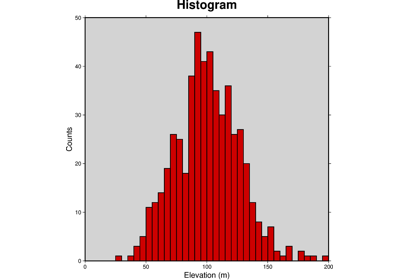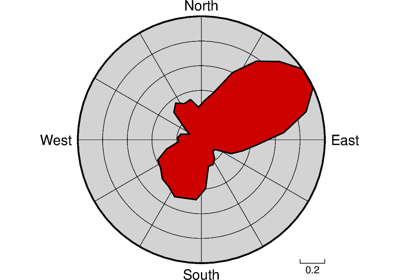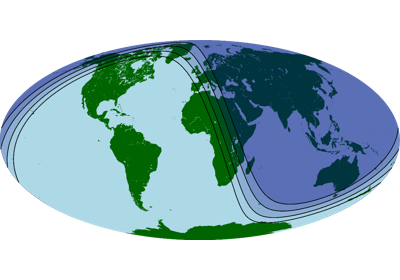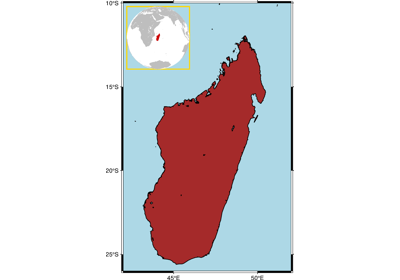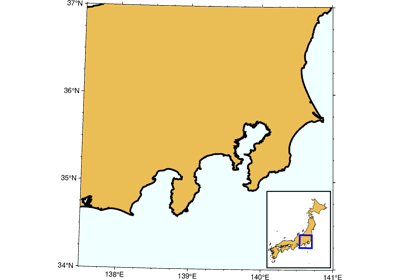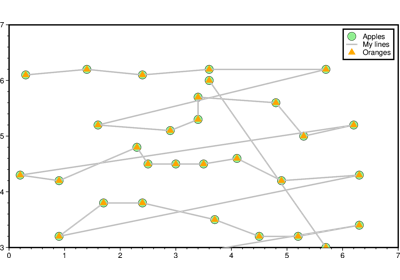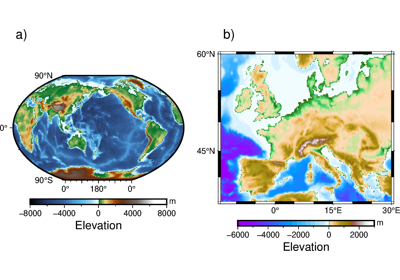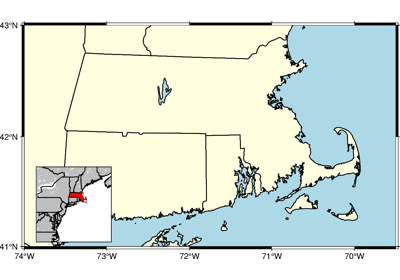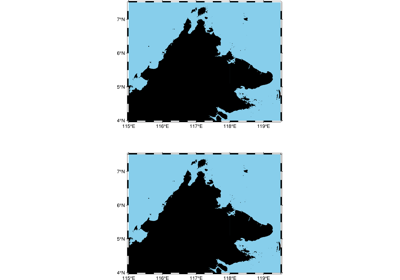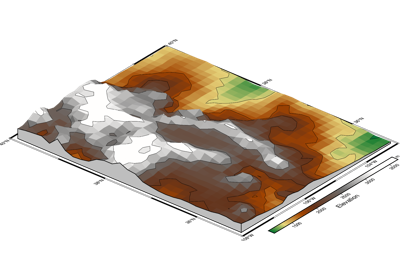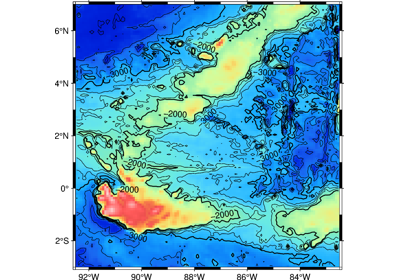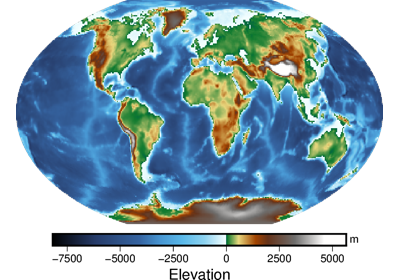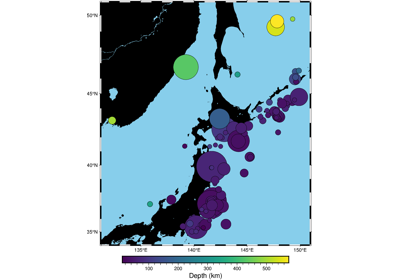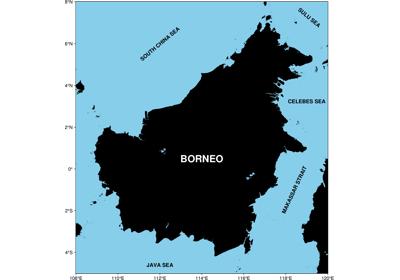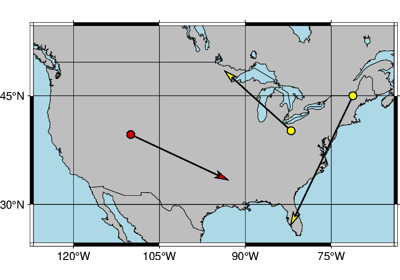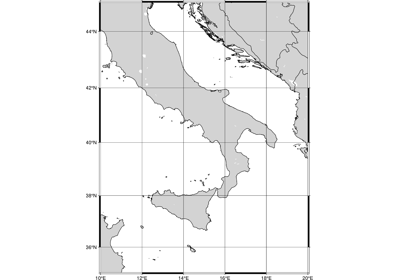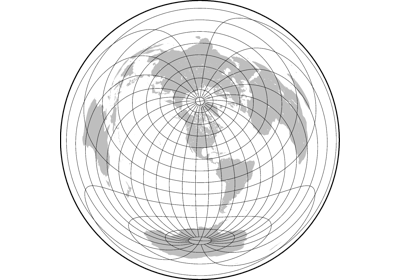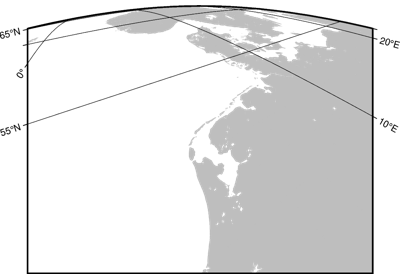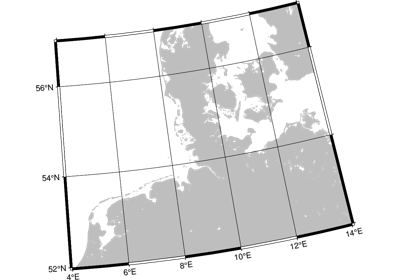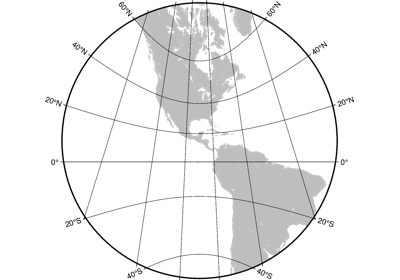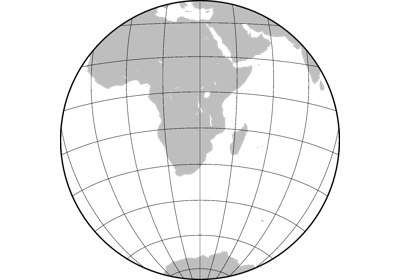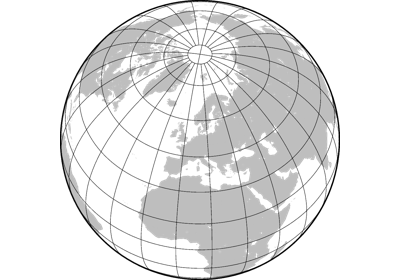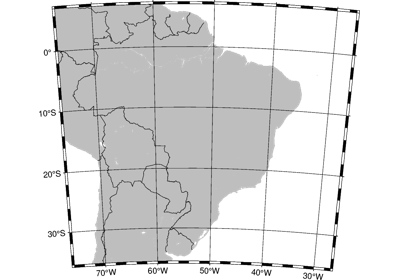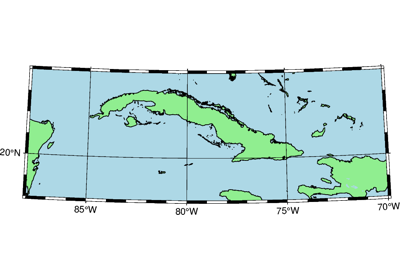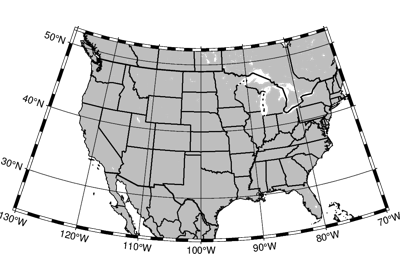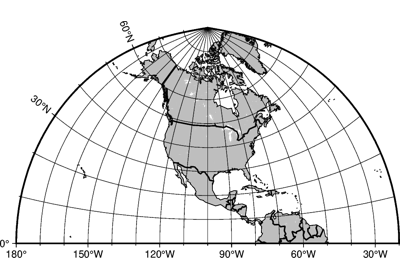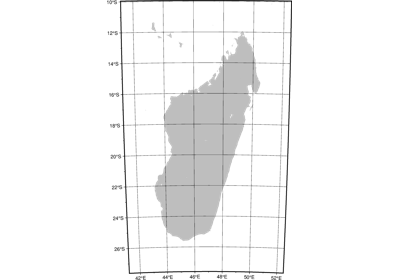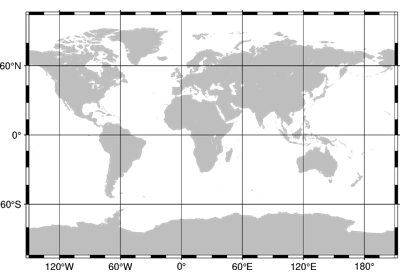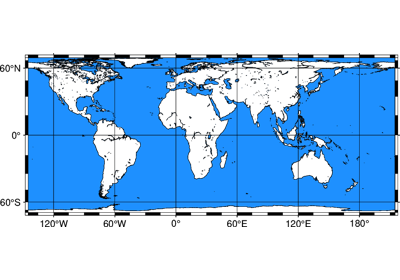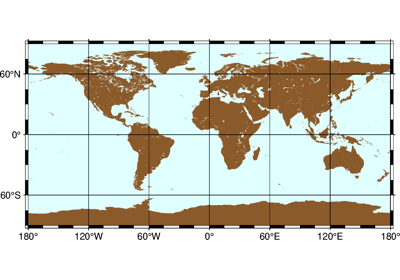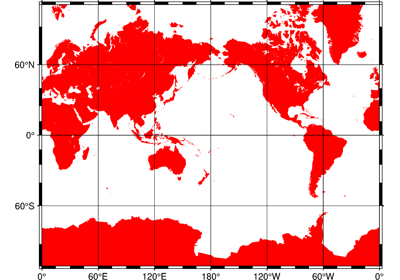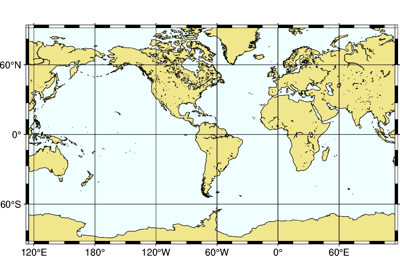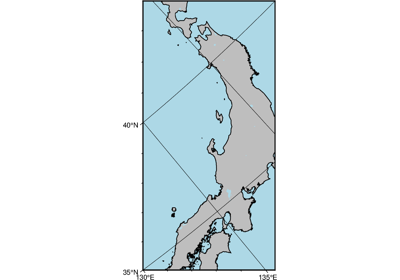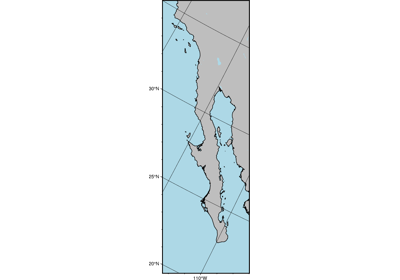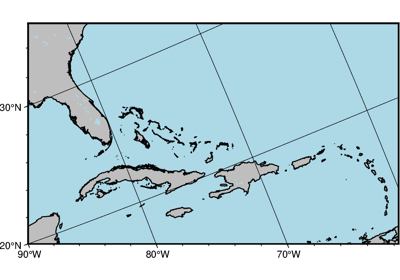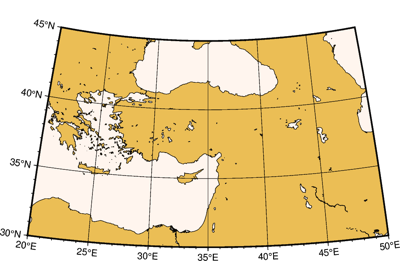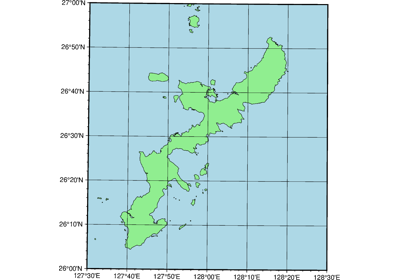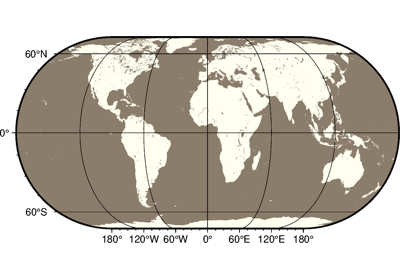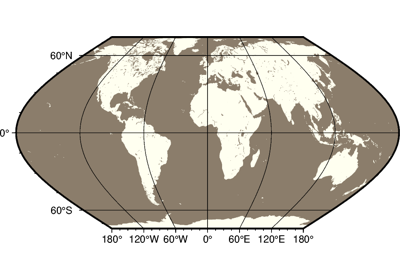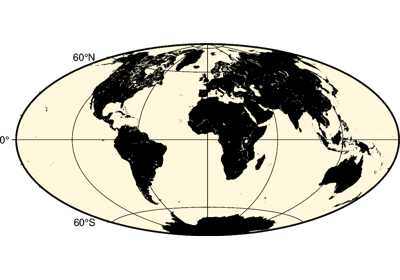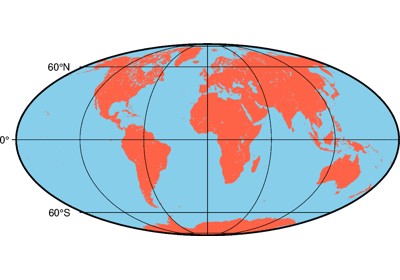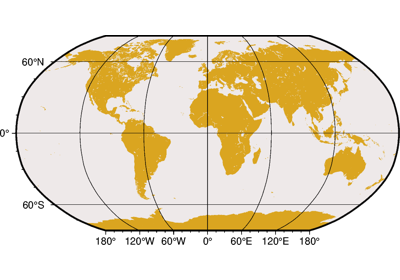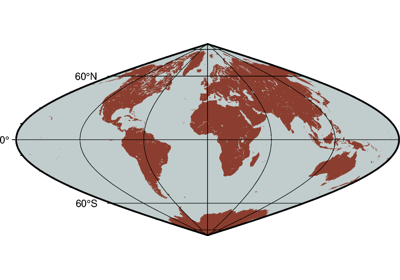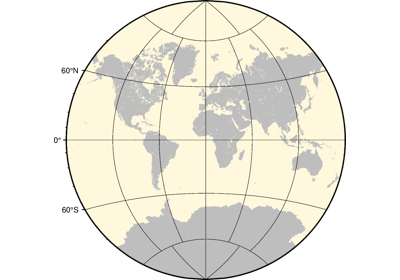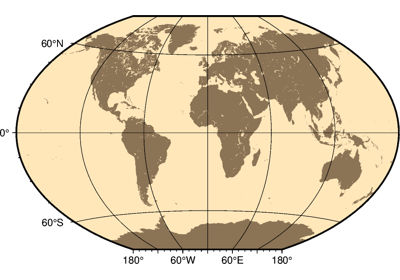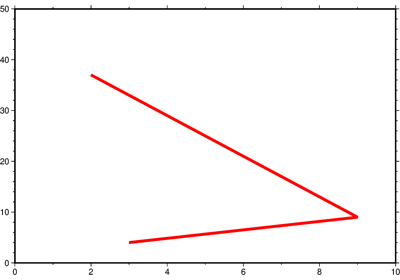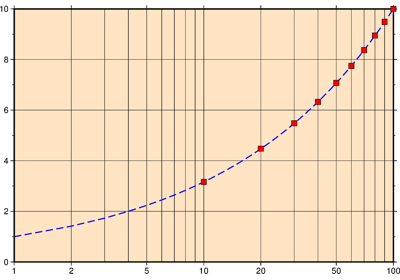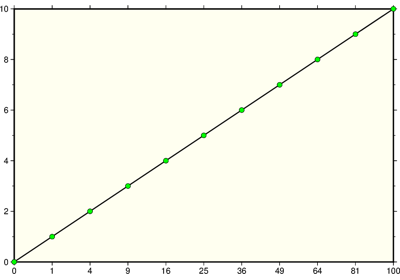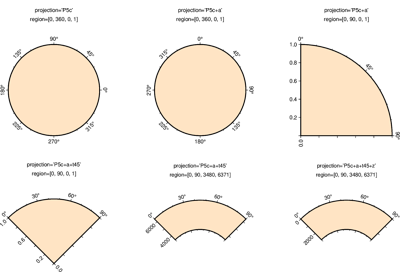# pygmt.Figure¶

class pygmt.Figure[source]

A GMT figure to handle all plotting.

Use the plotting methods of this class to add elements to the figure. You can preview the figure using pygmt.Figure.show and save the figure to a file using pygmt.Figure.savefig.

Unlike traditional GMT figures, no figure file is generated until you call pygmt.Figure.savefig or pygmt.Figure.psconvert.

Examples

>>> fig = Figure()
>>> fig.basemap(region=[0, 360, -90, 90], projection="W7i", frame=True)
>>> fig.savefig("my-figure.png")
>>> # Make sure the figure file is generated and clean it up
>>> import os
>>> os.path.exists("my-figure.png")
True
>>> os.remove("my-figure.png")


The plot region can be specified through ISO country codes (for example, 'JP' for Japan):

>>> fig = Figure()
>>> fig.basemap(region="JP", projection="M3i", frame=True)
>>> # The fig.region attribute shows the WESN bounding box for the figure
>>> print(", ".join("{:.2f}".format(i) for i in fig.region))
122.94, 145.82, 20.53, 45.52


Methods Summary

 Figure.basemap(*[, region, projection, …]) Plot base maps and frames for the figure. Figure.coast(*[, region, projection, …]) Plot continents, shorelines, rivers, and borders on maps Figure.colorbar(*[, region, projection, …]) Plot a gray or color scale-bar on maps. Figure.contour([x, y, z, data, annotation, …]) Contour table data by direct triangulation. Figure.grdcontour(grid, *[, annotation, …]) Convert grids or images to contours and plot them on maps. Figure.grdimage(grid, *[, img_out, frame, …]) Project and plot grids or images. Figure.grdview(grid, *[, region, …]) Create 3-D perspective image or surface mesh from a grid. Figure.histogram(table, *[, horizontal, …]) Plots a histogram, and can read data from a file or list, array, or dataframe. Figure.image(imagefile, *[, region, …]) Place images or EPS files on maps. Figure.inset(*[, position, box, margin, …]) Create an inset figure to be placed within a larger figure. Figure.legend([spec, position, box, region, …]) Plot legends on maps. Figure.logo(*[, region, projection, …]) Plot the GMT logo. Figure.meca(spec, scale[, longitude, …]) Plot focal mechanisms. Figure.plot([x, y, data, size, direction, …]) Plot lines, polygons, and symbols in 2-D. Figure.plot3d([x, y, z, data, size, …]) Plot lines, polygons, and symbols in 3-D. Figure.psconvert(*[, crop, gs_option, dpi, …]) Convert [E]PS file(s) to other formats. Figure.rose([length, azimuth, data, sector, …]) Plot windrose diagrams or polar histograms. Figure.savefig(fname[, transparent, crop, …]) Save the figure to a file. Figure.set_panel([panel, fixedlabel, …]) Set the current subplot panel to plot on. Figure.shift_origin([xshift, yshift]) Shift plot origin in x and/or y directions. Figure.show([dpi, width, method]) Display a preview of the figure. Figure.solar([terminator, …]) Plot day-light terminators or twilights. Figure.subplot([nrows, ncols, figsize, …]) Create multi-panel subplot figures. Figure.text([textfiles, x, y, position, …]) Plot or typeset text strings of variable size, font type, and orientation. Figure.velo([data, vector, frame, cmap, …]) Plot velocity vectors, crosses, anisotropy bars, and wedges. Figure.wiggle([x, y, z, data, frame, …]) Plot z=f(x,y) anomalies along tracks.

## Examples using pygmt.Figure¶